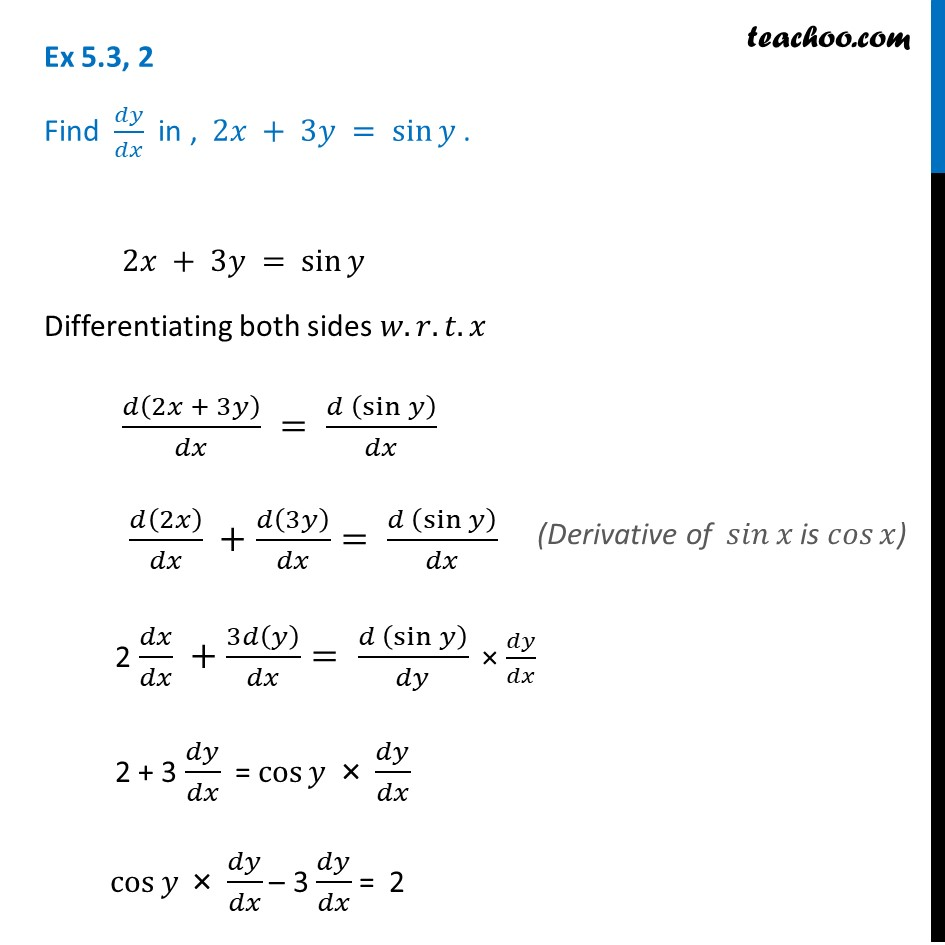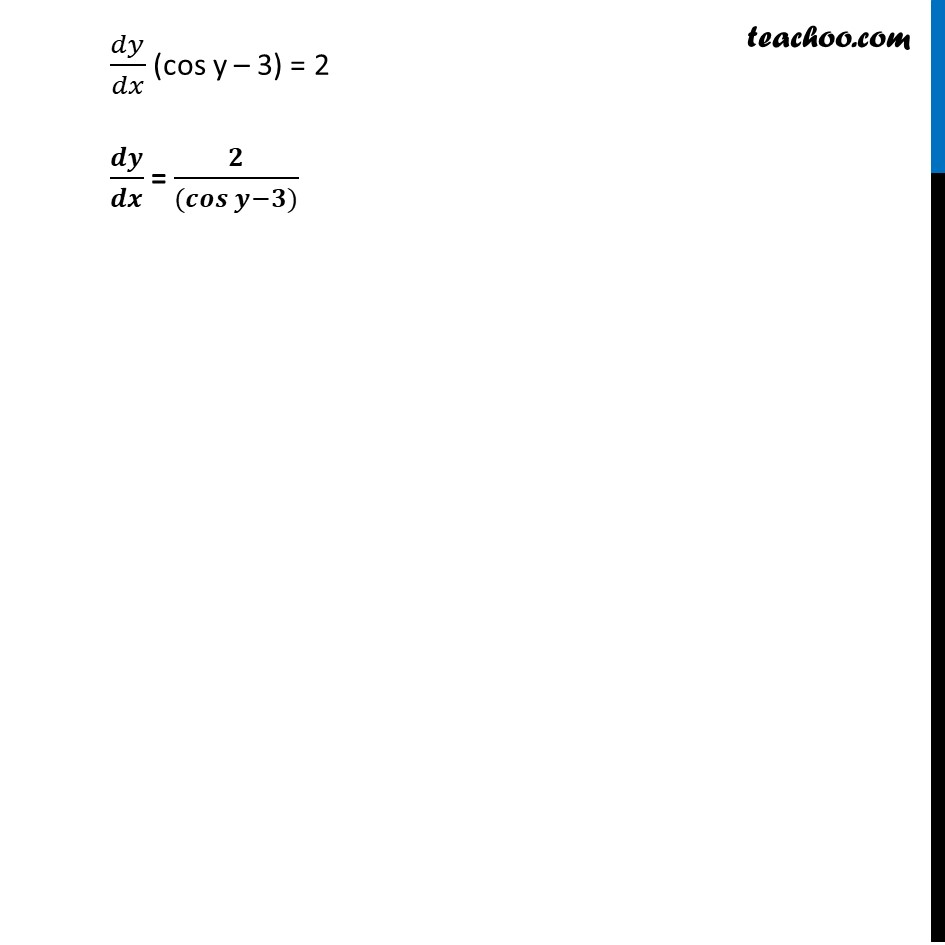Finding derivative of Implicit functions

Chapter 5 Class 12 Continuity and Differentiability
Concept wiseIntroducing your new favourite teacher - Teachoo Black, at only ₹83 per month

### Transcript

Ex 5.3, 2 Find 𝑑𝑦/𝑑𝑥 in , 2𝑥 + 3𝑦 = sin⁡𝑦 . 2𝑥 + 3𝑦 = sin⁡𝑦 Differentiating both sides 𝑤.𝑟.𝑡.𝑥 𝑑(2𝑥 + 3𝑦)/𝑑𝑥 = (𝑑 (sin⁡𝑦 ))/𝑑𝑥 𝑑(2𝑥)/𝑑𝑥 +𝑑(3𝑦)/𝑑𝑥= (𝑑 (sin⁡𝑦 ))/𝑑𝑥 2 𝑑𝑥/𝑑𝑥 +3𝑑(𝑦)/𝑑𝑥= (𝑑 (sin⁡𝑦 ))/𝑑𝑦 × 𝑑𝑦/𝑑𝑥 2 + 3 𝑑𝑦/𝑑𝑥 = cos⁡𝑦 "× " 𝑑𝑦/𝑑𝑥 cos⁡𝑦 "× " 𝑑𝑦/𝑑𝑥 – 3 𝑑𝑦/𝑑𝑥 = 2 (Derivative of 𝑠𝑖𝑛⁡𝑥 is 𝑐𝑜𝑠⁡𝑥) 𝑑𝑦/𝑑𝑥 (cos y – 3) = 2 𝒅𝒚/𝒅𝒙 = 𝟐/((𝒄𝒐𝒔⁡〖𝒚−𝟑)〗 )